Ex 12.2

Chapter 12 Class 7 Symmetry
Serial order wise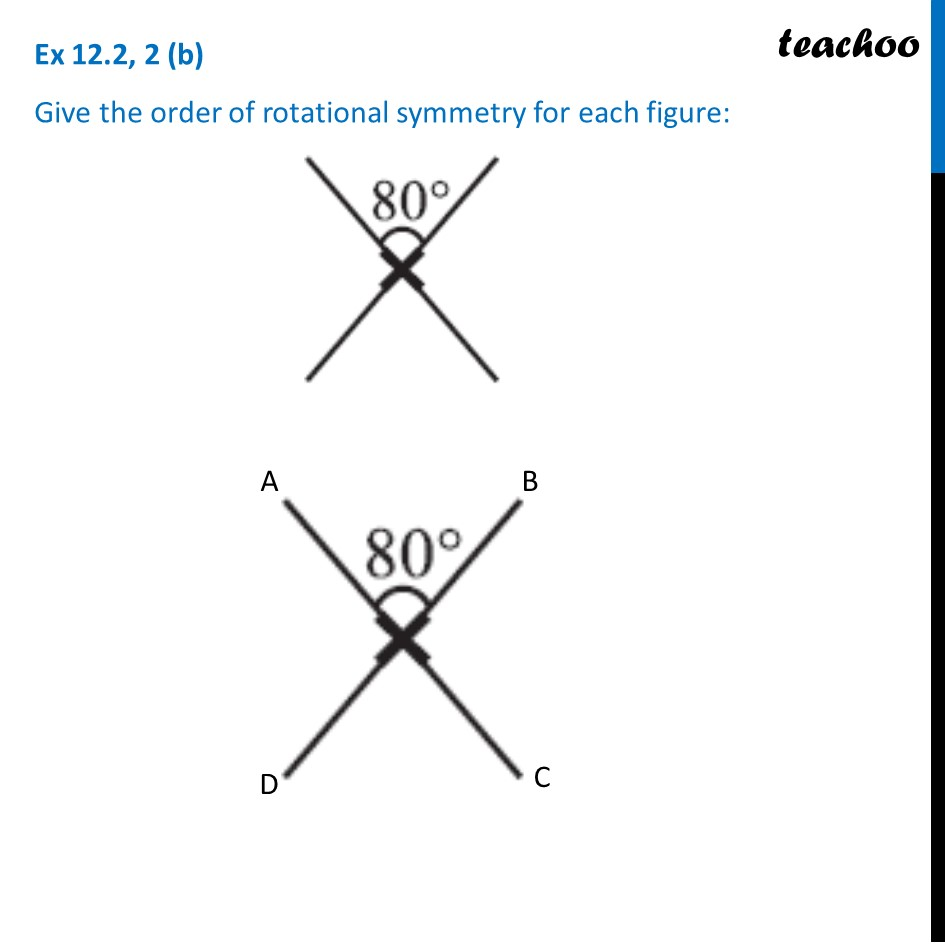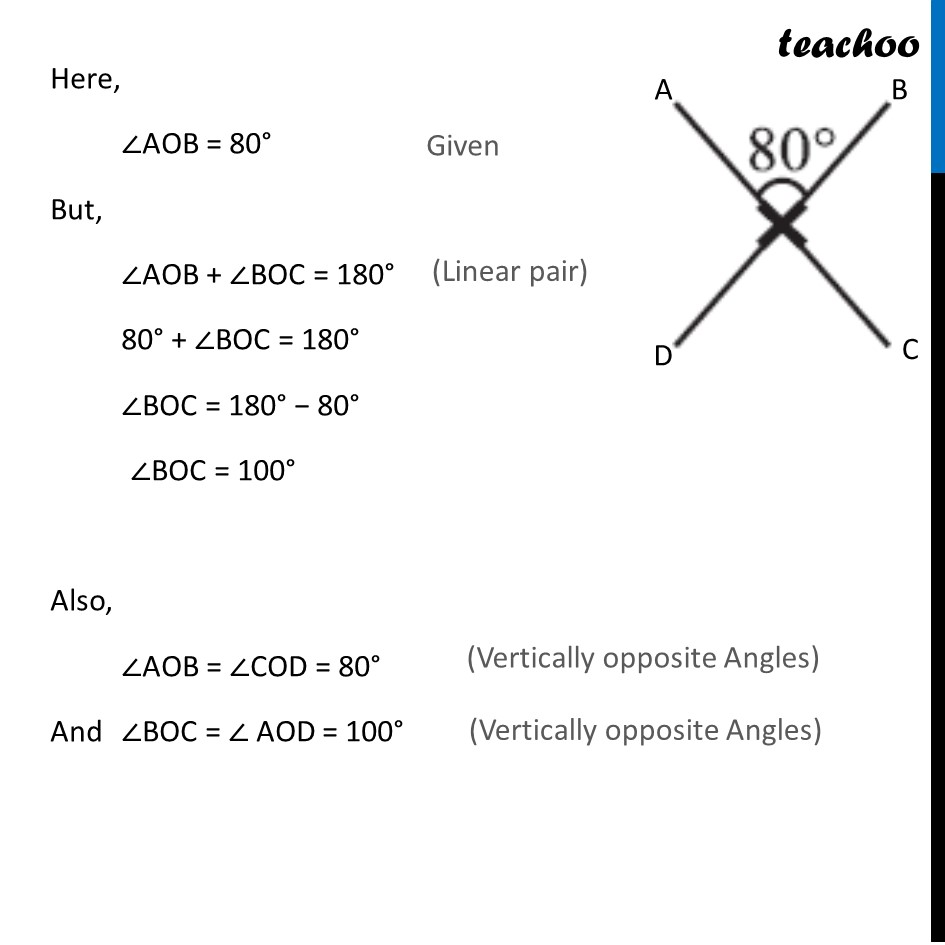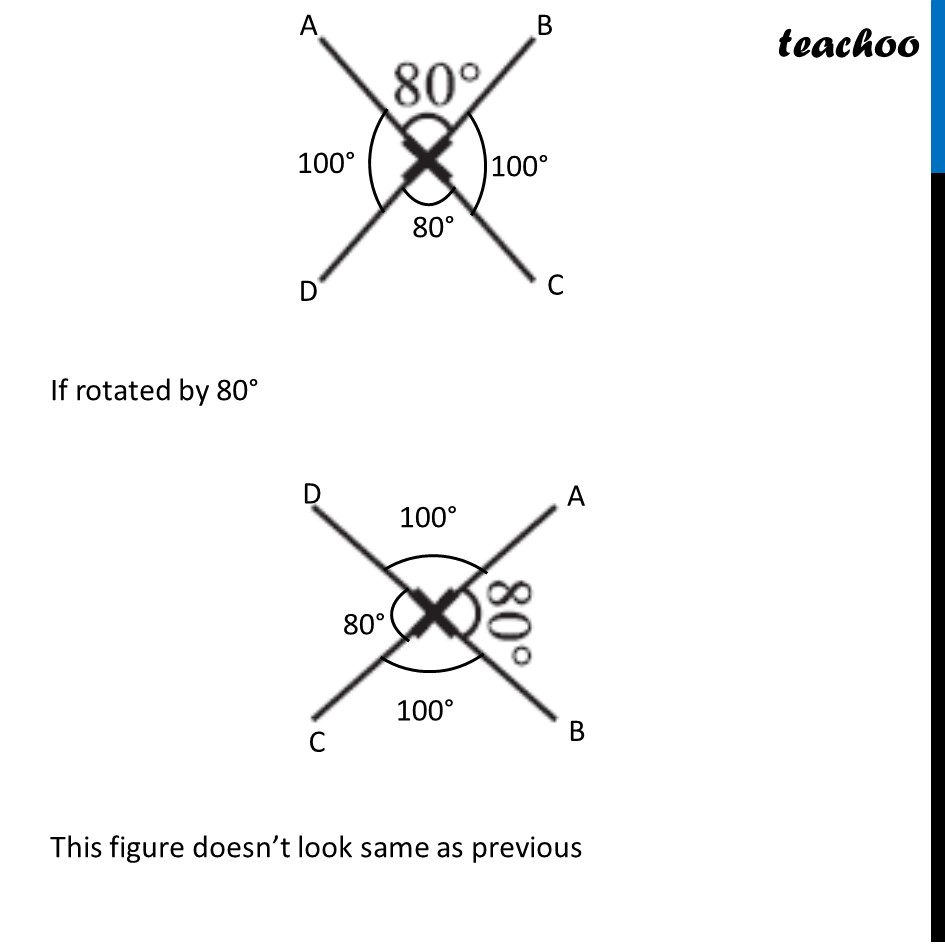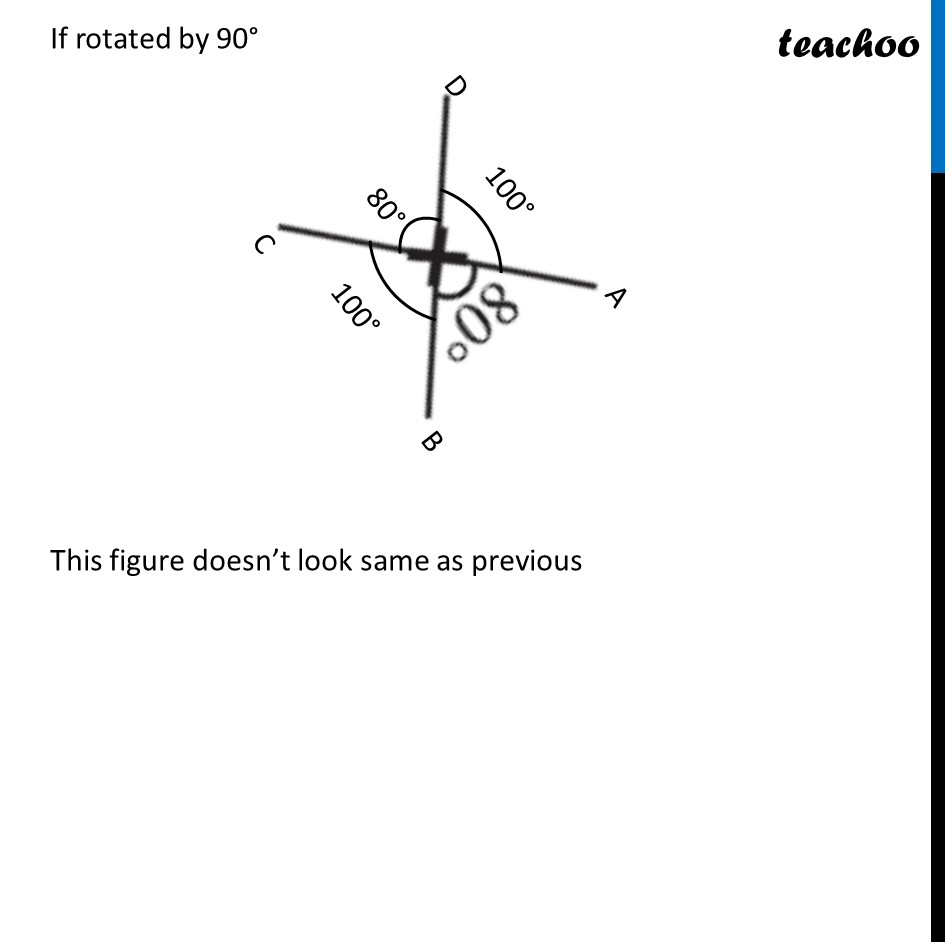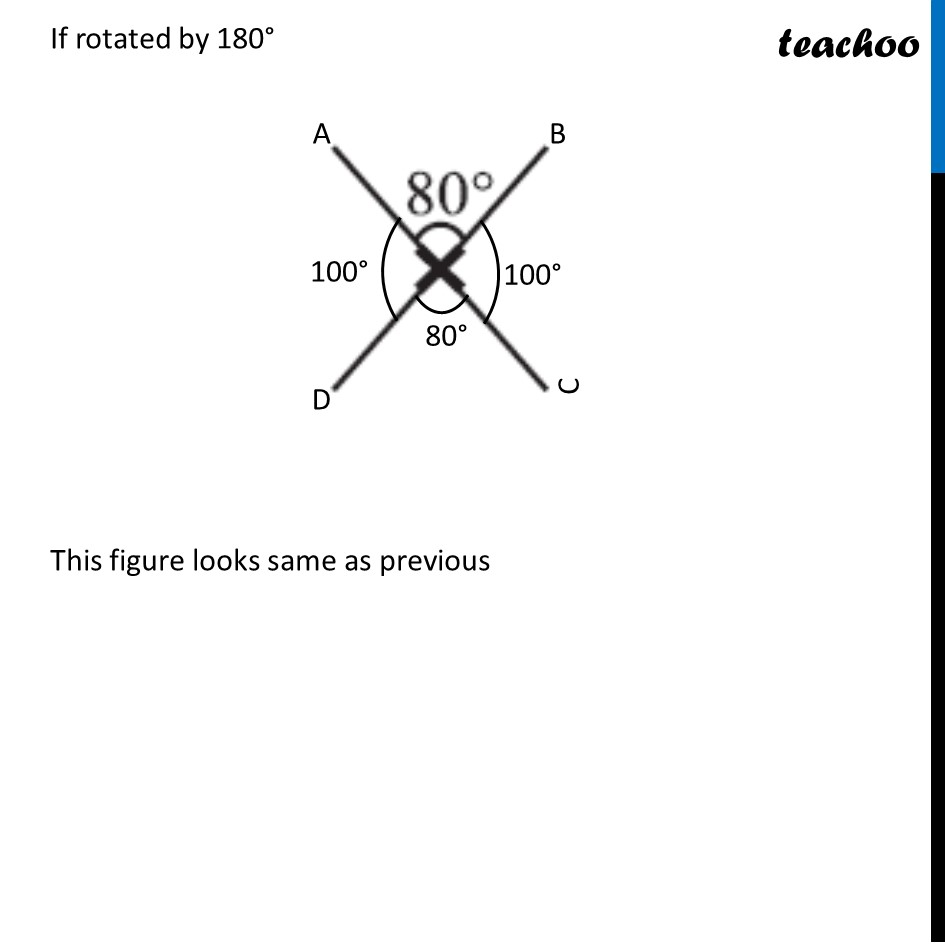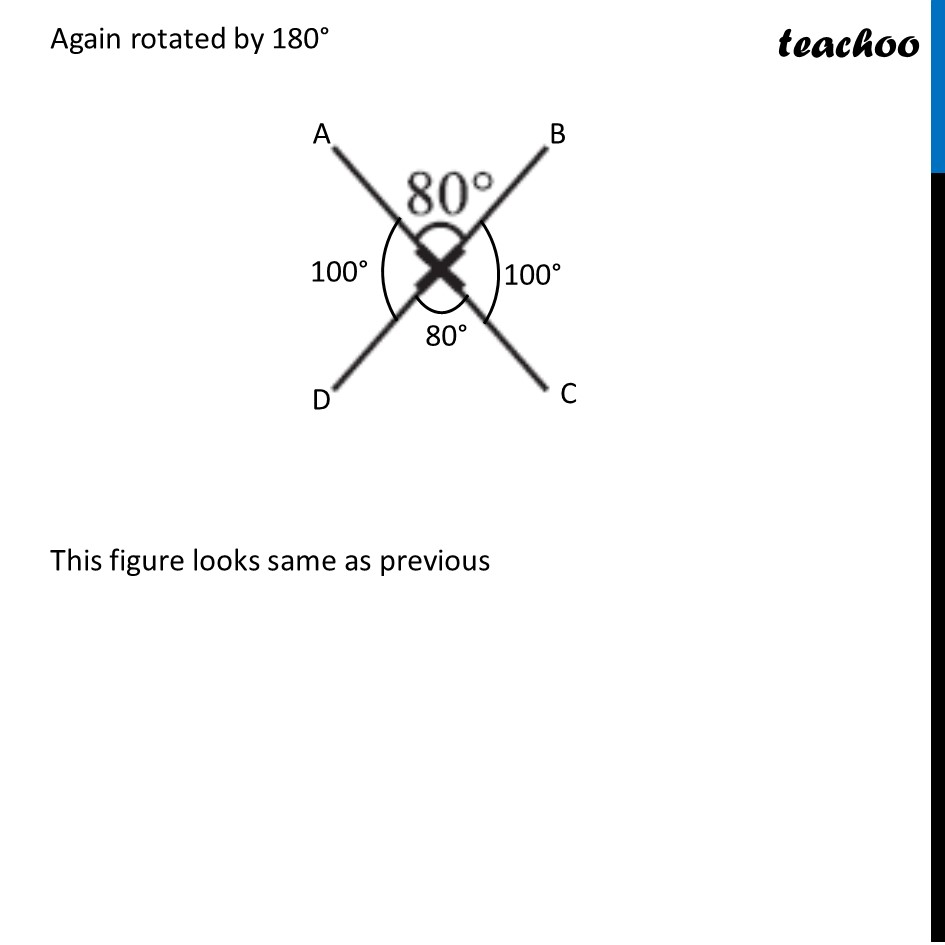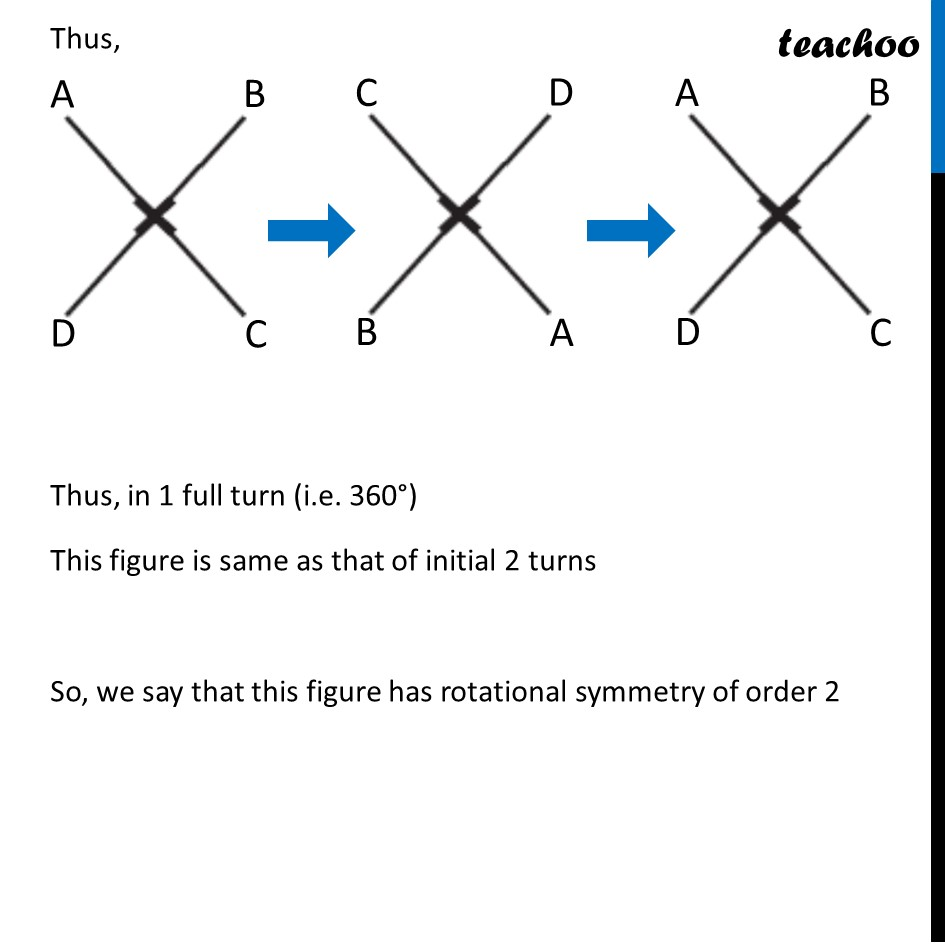Learn in your speed, with individual attention - Teachoo Maths 1-on-1 Class

### Transcript

Ex 12.2, 2 (b) Give the order of rotational symmetry for each figure: Here, ∠AOB = 80° But, ∠AOB + ∠BOC = 180° 80° + ∠BOC = 180° ∠BOC = 180° − 80° ∠BOC = 100° Also, ∠AOB = ∠COD = 80° And ∠BOC = ∠ AOD = 100° Thus, Thus, in 1 full turn (i.e. 360°) This figure is same as that of initial 2 turns So, we say that this figure has rotational symmetry of order 2